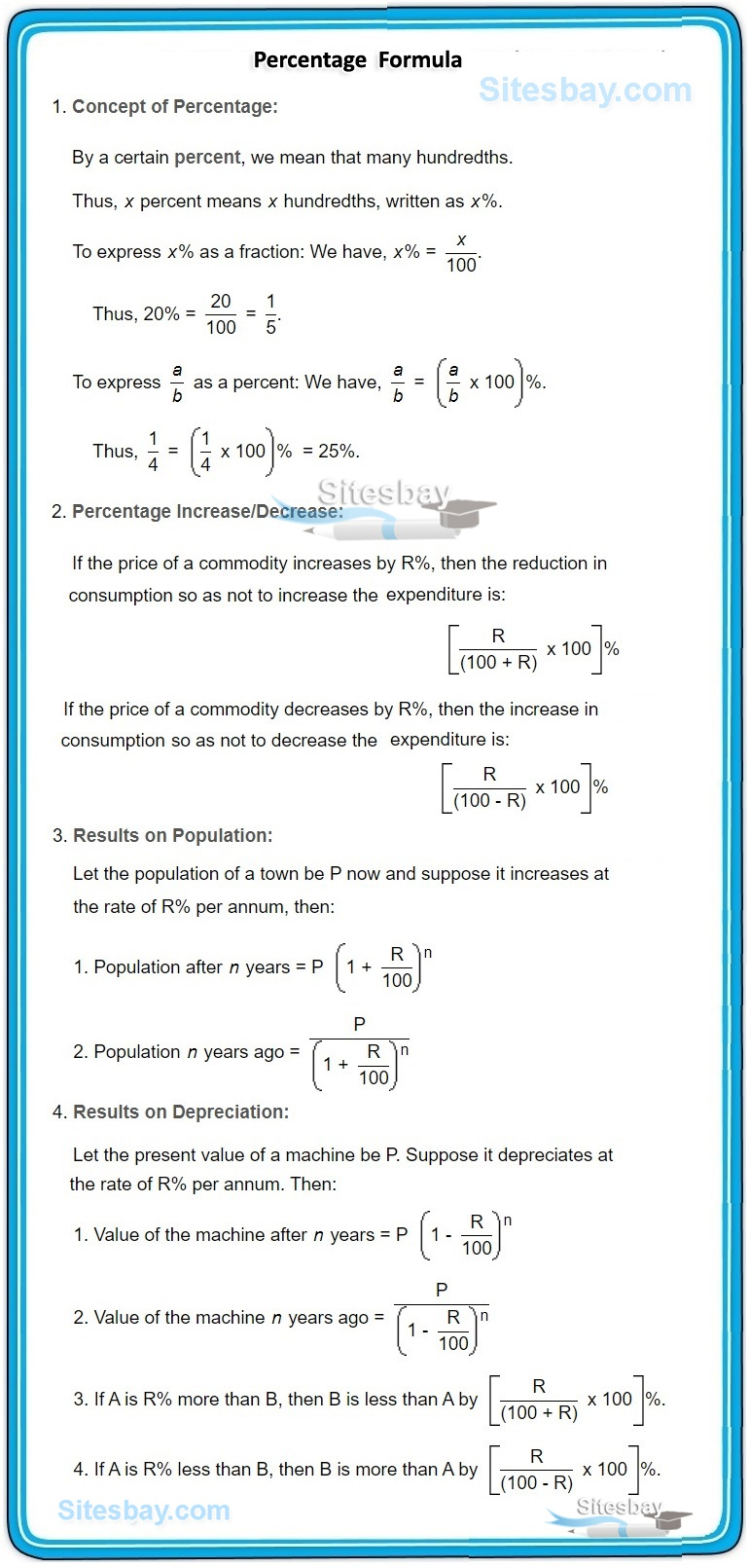# Percentage Aptitude Problems

## Percentage Aptitude Problems Tips and Tricks

In mathematics, a Percentage is a number or ratio that represents a fraction of 100. It is often denoted by the symbol "%" or simply as "percent" or "pct." For example, 35% is equivalent to the decimal 0.35, or the fraction.Rule : If x % of a quantity is taken by the first person, y % of the remaining quantity is taken by second person and z % of the remaning is taken by third person. If P amount is left in the balance, then original amount Q will be.

## Solution

```Q = (P * 100 * 100 * 100) / (100-X) (100-Y) (100-Z)
```

If x % of a quantity is added, again y % of the increased quantity is added, again z % of the increased quantity is added, now it becomes P then the origial quantity Q is given by

## Solution

```Q = (P * 100 * 100 * 100) / (100+X) (100+Y) (100+Z)
```

If P is the original population of a town and annual increase is r % then Population after one year will be

## Solution

```P + Pr/100 - P(1+ r/100)
```

Question 1: The total population of a city is 6500.The number of males and females increases by 5 % and 10 % respectively and consequently the population becomes 7000. Find the number of males in the village.

• (A) 4000
• (B) 3000
• (C) 3500
• (D) 2950

## Solution

```We are given that,
1) Total population of city = 6500
2) Increase in male and female population = 5 % & 10% respectively.
3) Final population of city = 7000
Hence,
Let's assume that number of males = x
Number of female = 6500 – x
Therefore, after increase in 5 % male and 10 % female, the population becomes 7000
5 % male +10 % female = Difference between new and original population

(5/100)*x  + 10/100(6500 – x) = 7000 – 6500
5x+65000-10x=50000
5x= 15000
x=3000
Number of males = 3000
Number of females = 3500
```

Question 2: A fruit seller had some apples. He sells 40% apples and still has 420 apples. Originally, he had:

• (A) 588 apples
• (B) 600 apples
• (C) 672 apples
• (D) 700 apples

## Solution

```Suppose originally he had x apples.
Then, (100 - 40)% of x = 420.
=60/100*X=420
X=(420*100)/60=700
```

Question 1: What percentage of numbers from 1 to 70 have 1 or 9 in the unit's digit?

• (A) 1
• (B) 14
• (C) 20
• (D) 21

## Solution

```Clearly, the numbers which have 1 or 9 in the unit's digit,
have squares that end in the digit 1. Such numbers from 1 to 70
are 1, 9, 11, 19, 21, 29, 31, 39, 41, 49, 51, 59, 61, 69.

Number of such number =14
Required percentage = (14/70)*100 = 20%
```

Question 3: The population of a town increased from 1,75,000 to 2,62,500 in a decade. The average percent increase of population per year is:

• (A) 4.37%
• (B) 5%
• (C) 6%
• (D) 8.75%

## Solution

```Increase in 10 years = (262500 - 175000) = 87500.
Increase% = (87500/175000)*100 = 50%
Required average = (50/10) = 5%
```

Question 4: In a certain school, 20% of students are below 8 years of age. The number of students above 8 years of age is of the number of students of 8 years of age which is 48. What is the total number of students in the school?

• (A) 150
• (B) 20
• (C) 120
• (D) 100

## Solution

```Let the number of students be x. Then,

Number of students above 8 years of age = (100 - 20)% of x = 80% of x.

80% of x = 48 + 2/3 of 48
80/100 X = 80
X = 100
```